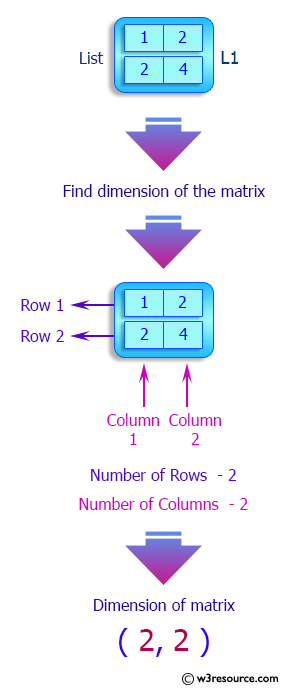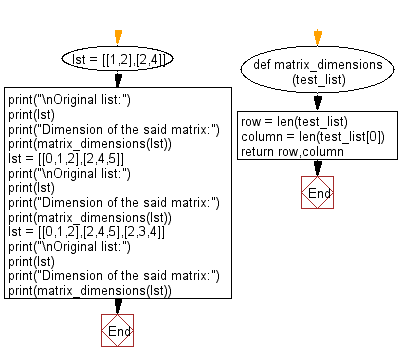﻿ Python: Find the dimension of a given matrix - w3resource# Python: Find the dimension of a given matrix

## Python List: Exercise - 193 with Solution

Write a Python program to find the dimension of a given matrix.

Sample Solution:

Python Code:

``````def matrix_dimensions(test_list):
row = len(test_list)
column = len(test_list)
return row,column

lst = [[1,2],[2,4]]
print("\nOriginal list:")
print(lst)
print("Dimension of the said matrix:")
print(matrix_dimensions(lst))
lst = [[0,1,2],[2,4,5]]
print("\nOriginal list:")
print(lst)
print("Dimension of the said matrix:")
print(matrix_dimensions(lst))
lst = [[0,1,2],[2,4,5],[2,3,4]]
print("\nOriginal list:")
print(lst)
print("Dimension of the said matrix:")
print(matrix_dimensions(lst))
```
```

Sample Output:

```Original list:
[[1, 2], [2, 4]]
Dimension of the said matrix:
(2, 2)

Original list:
[[0, 1, 2], [2, 4, 5]]
Dimension of the said matrix:
(2, 3)

Original list:
[[0, 1, 2], [2, 4, 5], [2, 3, 4]]
Dimension of the said matrix:
(3, 3)
```

Pictorial Presentation:Flowchart:## Visualize Python code execution:

The following tool visualize what the computer is doing step-by-step as it executes the said program:

Python Code Editor:

Have another way to solve this solution? Contribute your code (and comments) through Disqus.

What is the difficulty level of this exercise?

Test your Python skills with w3resource's quiz

﻿

## Python: Tips of the Day

```print(2_000_000)
```2000000